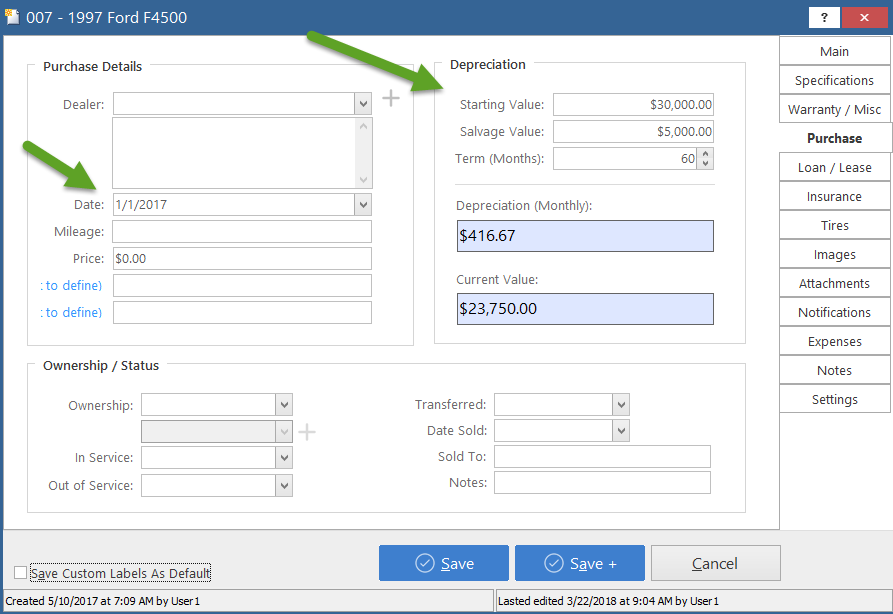# Depreciation Calculation

You can configure an asset's depreciation settings by going to your Equipment List and editing a piece of equipment/asset.

Once you are in the Edit screen, click on  Purchase on the right hand side. You will see this window appear:We will go over the example above to determine how our depreciation is calculated.

On the left hand side under Purchase Details you will see a  Date box. This is the date this particular vehicle was purchased, and when depreciation calculations begin. On the right hand side you will see a Depreciation section. We will go over each item in detail:

Starting Value: This is how much the vehicle was worth upon purchase.

Salvage Value: This is how much the vehicle will be worth no matter how much time has passed. The Current Value will never go below Salvage Value.

Term (Months): This is how long it will take for the car to reach its Salvage Value. In the example above, we are assuming that our \$30,000 vehicle will be worth \$5,000 at resale after 60 months.

Depreciation (Monthly): The calculation for this box is as follows.

\$30,000 Starting value - \$5,000 Salvage value = \$25,000 Depreciation overall
We take the \$25,000 depreciation and fit it into the 60 month term.
\$25,000 total depreciation divided by 60 months = \$416.66 depreciation per month

Current Value: This is obtained by taking your Starting Value of \$30,000 and combining it with your Date of purchase. Since the date was in January  of 2017, and the screenshot above was taken in March of 2018, the software will calculate us being 15 months from the purchase date (March is counted as one month even though March isn't technically finished yet). Our calculation is as follows:

\$30,000 Starting Value - (\$416.67 depreciation per month multiplied by 15 months, which equals very close to \$6250) = \$23,750.00 of value left on the vehicle after depreciation

In the Reports section of our software, all depreciation reports will pull from the values on the screenshot above.